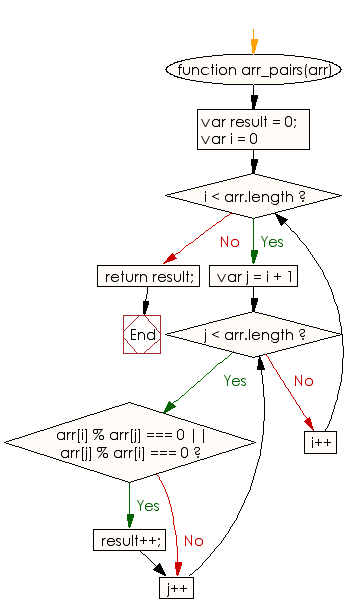# JavaScript: Find the number of sorted pairs formed by its elements of a given array of integers such that one element in the pair is divisible by the other one

## JavaScript Basic: Exercise-107 with Solution

Write a JavaScript program to find the number of sorted pairs formed by its elements of a given array of integers such that one element in the pair is divisible by the other one.

For example - The output of [1, 3, 2] ->2 - (1,3), (1,2).
The output of [2, 4, 6] -> 2 - (2,4), (2,6)
The output of [2, 4, 16] -> 3 - (2,4), (2,16), (4,16)

Sample Solution:

HTML Code:

``````<!DOCTYPE html>
<html>
<meta charset="utf-8">
<meta name="viewport" content="width=device-width">
<title> Find the number of sorted pairs formed by its elements of a given array of integers such that one element in the pair is divisible by the other one</title>
<body>

</body>
</html>
```
```

JavaScript Code:

``````function arr_pairs(arr) {
var result = 0;
for (var i = 0; i < arr.length; i++)
{
for (var j = i + 1; j < arr.length; j++)
{
if (arr[i] % arr[j] === 0 || arr[j] % arr[i] === 0)
{
result++;
}
}
}
return result;
}
console.log(arr_pairs([1,2,3]))
console.log(arr_pairs([2,4,6]))
console.log(arr_pairs([2,4,16]))
``````

Sample Output:

```2
2
3
```

Flowchart:ES6 Version:

``````function arr_pairs(arr) {
let result = 0;
for (let i = 0; i < arr.length; i++)
{
for (let j = i + 1; j < arr.length; j++)
{
if (arr[i] % arr[j] === 0 || arr[j] % arr[i] === 0)
{
result++;
}
}
}
return result;
}
console.log(arr_pairs([1,2,3]))
console.log(arr_pairs([2,4,6]))
console.log(arr_pairs([2,4,16]))
``````

Live Demo:

See the Pen javascript-basic-exercise-107 by w3resource (@w3resource) on CodePen.

What is the difficulty level of this exercise?

Test your Programming skills with w3resource's quiz.

﻿

## JavaScript: Tips of the Day

Converts a string to title case

Example:

```const toTitleCase = str =>
str
.match(/[A-Z]{2,}(?=[A-Z][a-z]+[0-9]*|\b)|[A-Z]?[a-z]+[0-9]*|[A-Z]|[0-9]+/g)
.map(x => x.charAt(0).toUpperCase() + x.slice(1))
.join(' ');
console.log(toTitleCase('some_database_field_name')); // 'Some Database Field Name'
console.log(toTitleCase('Some label that needs to be title-cased')); // 'Some Label That Needs To Be Title Cased'
console.log(toTitleCase('some-package-name')); // 'Some Package Name'
console.log(toTitleCase('some-mixed_string with spaces_underscores-and-hyphens')); // 'Some Mixed String With Spaces Underscores And Hyphens'
```

Output:

```"Some Database Field Name"
"Some Label That Needs To Be Title Cased"
"Some Package Name"
"Some Mixed String With Spaces Underscores And Hyphens"
```